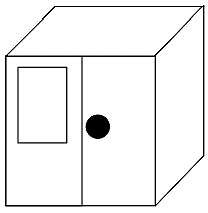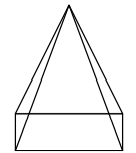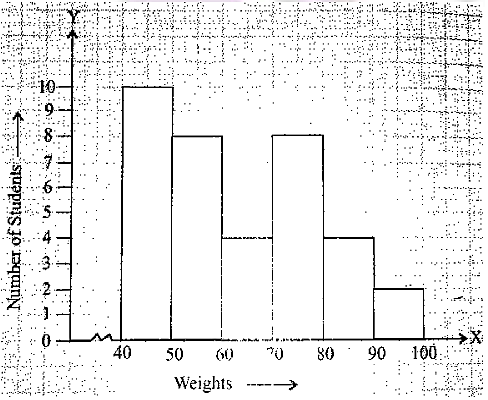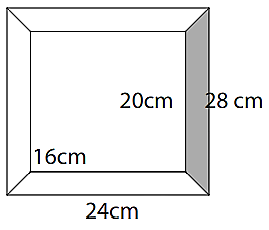### CBSE Class 8 Maths Sample Paper 3# CBSE Class 8 Maths Sample Paper 3

CBSE Class 8

Maths Sample Paper-3

Time Durations: 3:00 hrs.                                                                            Maximum Marks: 100

General Instructions:

• The question paper consists of 35 questions divided into 4 sections A, B, C and D. Section A comprises of 10 questions of 1 mark each. Section B comprises of 7 questions of 3 marks each. Section C comprises of 12 questions of 4 marks each. Section D comprises of 6 questions of 7 marks each.

• In Section – A all questions are compulsory. In Section – B solve any 5 questions. In Section – C solve any 10 questions and in Section – D solve any 5 questions.

• Draw neat diagrams wherever needed.

SECTION – A

(Attempt all questions)

1. The difference between the highest and the lowest values of a set of data is called________.

2. $text { The product of }(9-4),left(x^{4} yright),left(x y^{3}right) text { and }left(x^{3} y^{2}right) text { is }$ ______.

3. How many faces and edges does a triangular prism have?

4. TSA of a prism = LSA + 2x ______.

5. Write 0.0000507 in standard form.

6. $text { A pipe that fills } 25 % text { of a tank in } 1 text { hour will fill it completely in }$ ______ hours.

7. $4 x^{2}-9 y^{2}=?$

8. A point with y coordinate zero will lie on ______ axis.

9. $text { If } 62 mathrm{y}^{3}$ is a multiple of 3, where y is a single digit then what should be the minimum and maximum value of y?

10. A number divisible by both 2 and 5 must have ____ in its ones place

SECTION – B

(Attempt any five questions)

11. Evaluate using suitable identity: 1.05 × 9.5.

12. Simplify: 3y(2y – 7) – 3(y – 4) – 63 and evaluate for y = – 2.

13.  Draw the top, side and front view of the given figure14. Find the length of the altitude of a rhombus if lengths of its two diagonals are 12cm and 16cm respectively.

15. $text { Evaluate }:left(6^{-1}-7^{-1}right)^{-1}-left(5^{-1}-4^{-1}right)^{-1}$

16. Write Euler’s formula, then find the number of faces in a solid if the number of vertices is 8 and number of edges is 12.

17. In a stack there are 5 books each of thickness 20mm and 5 paper sheets each of thickness 0.016mm. What is the total thickness of the stack? Write in standard form.

SECTION – C

(Attempt any ten questions)

18. 12 cards numbered 1,2,3,……11,12 are kept in a box and mixed thoroughly. If one card is drawn at random, nd the probability of getting a card with:

i) a prime number                           ii) a factor of 12

iii) a number divisible by 3           iv) a multiple of 2

19. Evaluate without actual multiplication:

$text { (ii) }(105)^{2}$

$text { (ii) }(105)^{2}$

20. Simplify:

$text { (i) }(5 x-6)(2 x-3)+(3 x+5)^{2}$

$text { (ii) }(2 x+5 y)(2 x+3 y)$

21. Verify Euler’s formula for the given solid.22. Find the volume of a cube if its total surface area is 150cm2.

$text { (a) Find } mathrm{m} text { so that }(-3)^{mathrm{m}+1} times(-3)^{5}=(-3)^{7}$

$text { (b) Find the value of }left(3^{0}+4^{-1}right) times 2^{2}$

23. A 5m 60cm high pole casts a shadow of length 3m 20cm.

(a) Find at the same time the length of a shadow cast by another pole 10m 50cm high.

(b) Find the height of the pole if the length of the shadow is 6m 40cm.

24. Factorise:

$text { (a) } m^{4}-256$
$text { (b) } x^{2}+x y+8 x+8 y$

25. (a)$text{Find the highest common factor of } 16 x^{3},-4 x^{2}, 32 x$

(b)$text{Factorise } x^{2}-14 x+13$

26. Given below is the histogram showing the weights of 36 students of a hostel:(i) What is the class size?

(ii) How many students are there in the class intervals of weights 40-70 and 80-90?

(iii) How many students weigh 70 kg or more?

27. An aquarium is in the form of a cuboid whose external measures are 80 cm x 30 cm x 40 cm. The base, side faces and back face are to be covered with the coloured paper. Find the area of paper needed.

28. Work out the following divisions:

$text { (i) }left(7 x^{2}+14 xright) div(x+2)$
$text { (ii) } 5 p qleft(p^{2}-q^{2}right) div 2 p(p+q)$

SECTION – D

(Attempt any five questions)

29. On a particular day, the sales (in rupees) of different items of a baker’s shop are given below. Draw a pie chart for this data:

$begin{array}{|l|l|}hline text { Ordinary bread } & 320 \hline text { Fruit } & 80 \hline text { Cakes and pastries } & 160 \hline text { Biscuits } & 120 \hline text { Others } & 40 \hlinetext { TOTAL } & 720 \hlineend{array}$

30. Diagram of the given picture frame has outer dimensions as 24cm × 28cm and inner dimensions as 16cm × 20cm. Find the area of each section of the frame, if the width of each section is same.31. Rohit is making a wheel using spokes. He wants to -x equal spokes in such a way that the angles between any pair of consecutive spokes are equal. Help him by completing the following table:

$begin{array}{|c|c|c|c|c|}hline text { No. of Spokes } & 4 & 6 & 8 & 10 \hline text { Angle between a pair of consecutive spokes } & 90^{circ} & 60^{circ} & ? & ? \hlineend{array}$

(a) Are the number of spokes and the angles formed between the pair of consecutive spokes in inverse proportion?

(b) Calculate the angle between a pair of consecutive spokes on a wheel with 15 spokes.

(c) How many spokes would be needed if the angle between a pair of consecutive spokes is $40^{circ}$ ?

32. (a) $text{Factorise then divide :}frac{156left(36 y^{2}-64right) y^{3}}{104^{2}(6 y+8) y}$

(b) Factorise: $16mathrm{a}^{2}-25mathrm{~b}^{2}+60mathrm{bc}-36mathrm{c}^{2}$

33. Draw a line graph for the following

$begin{array}{|l|l|l|l|l|l|}hline text { Side of square(in } mathrm{cm}) & 10 & 20 & 25 & 30 & 40 \hline text { Perimeter (in cm) } & 40 & 80 & 100 & 120 & 160 \hlineend{array}$

34. (a) A milk tank is in the form of a cylinder whose radius is 1.5 m and length is 7m. Find the quantity of milk in litres that can be stored in the tank.

(b) Find the height of a cuboid whose volume is $275 mathrm{~cm}^{3}$ and base area is $25 mathrm{~cm}^{2}$

Privacy Settings